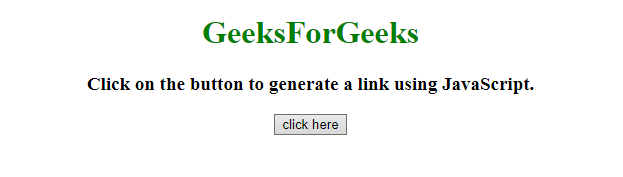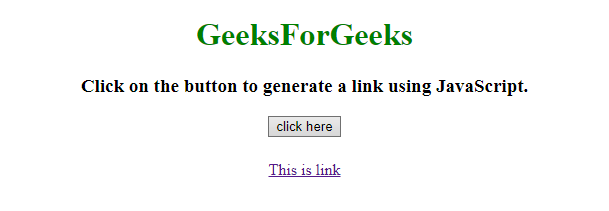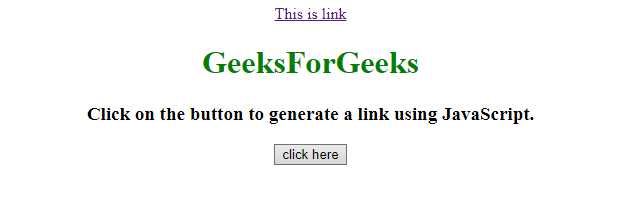# How to create a link in JavaScript ?

• Last Updated : 20 Jul, 2021

Given an HTML document and the task is to create a JavaScript link and add it to the document using JavaScript.

Approach:

Hey geek! The constant emerging technologies in the world of web development always keeps the excitement for this subject through the roof. But before you tackle the big projects, we suggest you start by learning the basics. Kickstart your web development journey by learning JS concepts with our JavaScript Course. Now at it's lowest price ever!

• Create an anchor <a> element.
• Create a text node with some text which will display as a link.
• Append the text node to the anchor <a> element.
• Set the title and href property of the <a> element.
• Append <a> element in the body.

Example 1: In this example, the node is created and the attributes are set by the JavaScript methods.

 ` ``<``html``> ``    ``<``head``> ``        ``<``title``> ``            ``How to create a link in JavaScript ?``        ````    `` ``     ` `    ``<``body` `style` `= ``"text-align:center;"``>``         ` `        ``<``h1` `style` `= ``"color:green;"` `> ``            ``GeeksForGeeks``        ````         ` `        ``<``p` `id` `= ``"GFG_UP"` `style` `=``            ``"font-size: 19px; font-weight: bold;"``>``        ````         ` `        ``<``button` `onclick` `= ``"GFG_Fun()"``>``            ``click here``        ````         ` `        ``<``p` `id` `= ``"GFG_DOWN"` `style` `=``            ``"color: green; font-size: 24px; font-weight: bold;"``>``        ````         ` `        ``<``script``>``            ``var el_up = document.getElementById("GFG_UP");``            ``var el_down = document.getElementById("GFG_DOWN");``             ` `            ``el_up.innerHTML = "Click on the button to generate "``                    ``+ "a link using JavaScript.";``             ` `            ``function GFG_Fun() {``                 ` `                ``// Create anchor element.``                ``var a = document.createElement('a'); ``                 ` `                ``// Create the text node for anchor element.``                ``var link = document.createTextNode("This is link");``                 ` `                ``// Append the text node to anchor element.``                ``a.appendChild(link); ``                 ` `                ``// Set the title.``                ``a.title = "This is Link"; ``                 ` `                ``// Set the href property.``                ``a.href = "https://www.geeksforgeeks.org"; ``                 ` `                ``// Append the anchor element to the body.``                ``document.body.appendChild(a); ``            ``}``        `` ``    `` ``                    `

Output:

• Before clicking on the button:• After clicking on the button:Example 2: This example is similar to the above but uses prepend() method to add anchor element to the body.

 ` ``<``html``> ``    ``<``head``> ``        ``<``title``> ``            ``How to create a link in JavaScript ?``        ````    `` ``     ` `    ``<``body` `style` `= ``"text-align:center;"``>``         ` `        ``<``h1` `style` `= ``"color:green;"` `> ``            ``GeeksForGeeks``        ````         ` `        ``<``p` `id` `= ``"GFG_UP"` `style` `=``            ``"font-size: 19px; font-weight: bold;"``>``        ````         ` `        ``<``button` `onclick` `= ``"GFG_Fun()"``>``            ``click here``        ````         ` `        ``<``p` `id` `= ``"GFG_DOWN"` `style` `=``            ``"color: green; font-size: 24px; font-weight: bold;"``>``        ````         ` `        ``<``script``>``            ``var el_up = document.getElementById("GFG_UP");``            ``var el_down = document.getElementById("GFG_DOWN");``             ` `            ``el_up.innerHTML = "Click on the button to generate "``                    ``+ "a link using JavaScript.";``             ` `            ``function GFG_Fun() {``                 ` `                ``// Create anchor element.``                ``var a = document.createElement('a'); ``                 ` `                ``// Create the text node for anchor element.``                ``var link = document.createTextNode("This is link");``                 ` `                ``// Append the text node to anchor element.``                ``a.appendChild(link); ``                 ` `                ``// Set the title.``                ``a.title = "This is Link"; ``                 ` `                ``// Set the href property.``                ``a.href = "https://www.geeksforgeeks.org"; ``                 ` `                ``// Append the anchor element to the body.``                ``document.body.prepend(a); ``            ``}``        `` ``    `` ``                    `

Output:

• Before clicking on the button:• After clicking on the button:JavaScript is best known for web page development but it is also used in a variety of non-browser environments. You can learn JavaScript from the ground up by following this JavaScript Tutorial and JavaScript Examples.

My Personal Notes arrow_drop_up